The Pareto principle is the 80-20 rule. It basically states that, roughly 80% of results come from 20% of the causes. For example, 80% of profits come from 20% of the products.

In Tableau, you can apply a table calculation to Profit data to create a chart that shows the percentage of total Profit that come from the top sub category. You can also see which subcategory account for 80% of total profit.

We will follow the steps to create a Pareto Chart in Tableau on sample Super Store Data set. We all know that Sample Superstore is a mock up data for a sample super store, so it may or may not be the case that the Data does not follow the Pareto Principle.

Let’s do it for the Sample Superstore data set that comes up with Tableau 10 and we will use Tableau Desktop 10.0 to validate if the Data set that we are looking into follows the Pareto Principle or not. So the Question that we are seeking an answer here is “Does 80% of the Profit is Contributed by 20% of the Products Sub Category

Step 1: Drag Subcategory onto Columns and Profit on to rows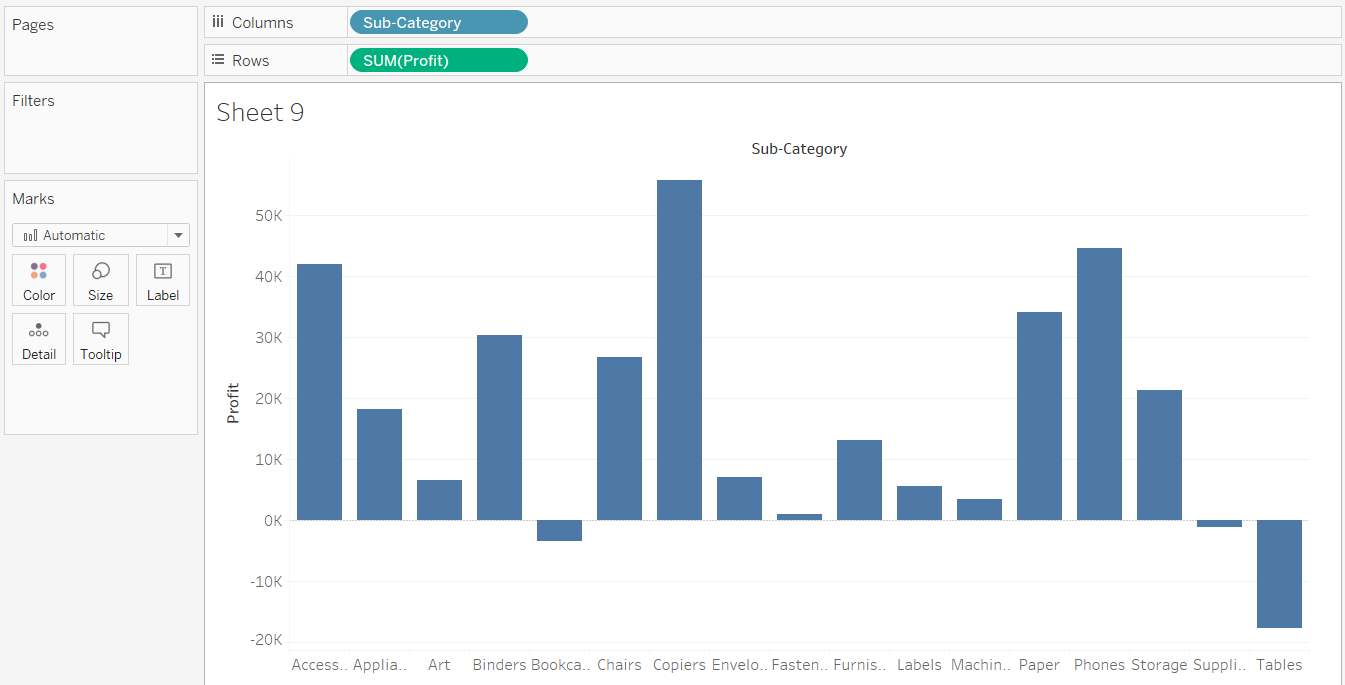Step 2: Sort in Descending Order on the basis of profit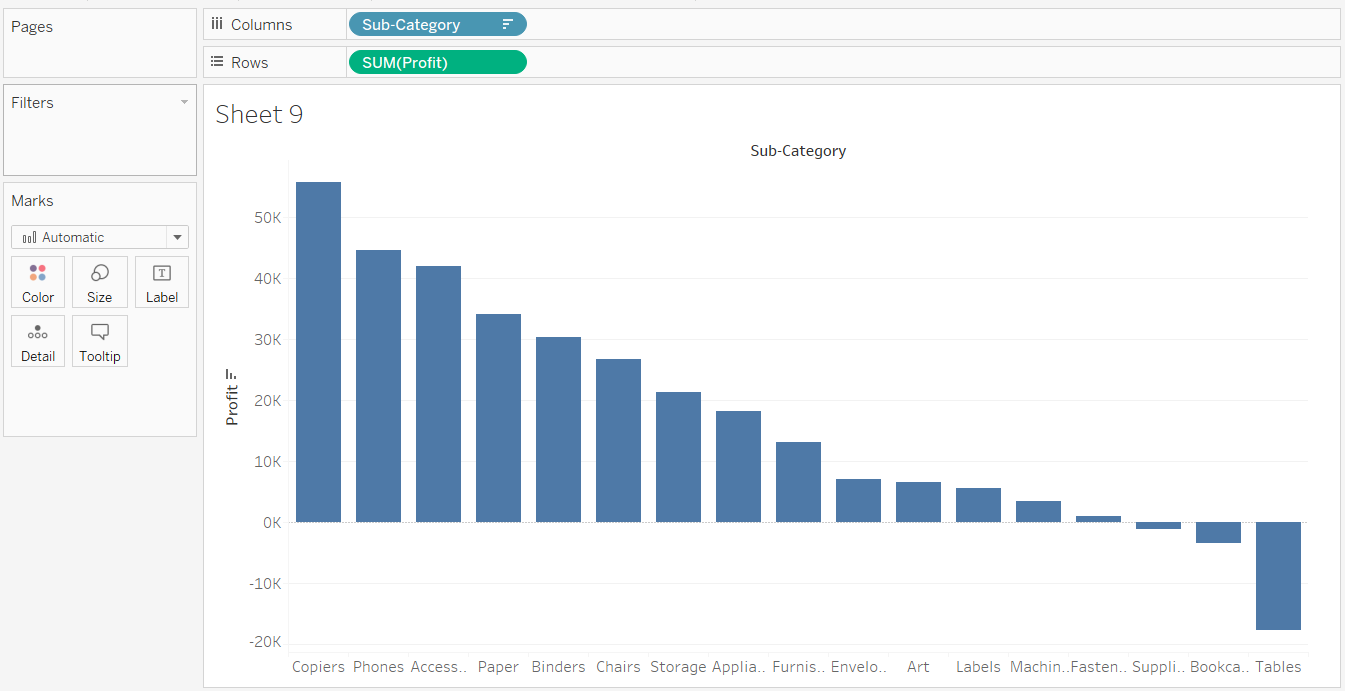Step 3: Add a Table Calculation (Running Total) of Sum of Profit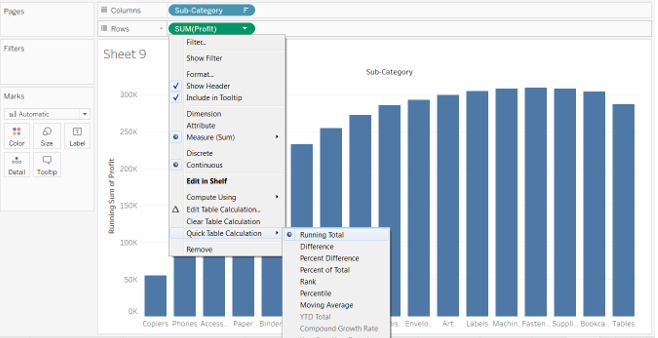So what we get till now is we have the “Running Total of Sum of Profit” i.e. Profit starts and the running total gives 100% of the profit that happened. So in order to know when 80% of the profit was done , we add a new secondary Table calculation on sum(profit) and change it to %.

Step 4: Change Running Total of Sum(Profit) from Actual Value to %. For this we add another Table calculation to it as below.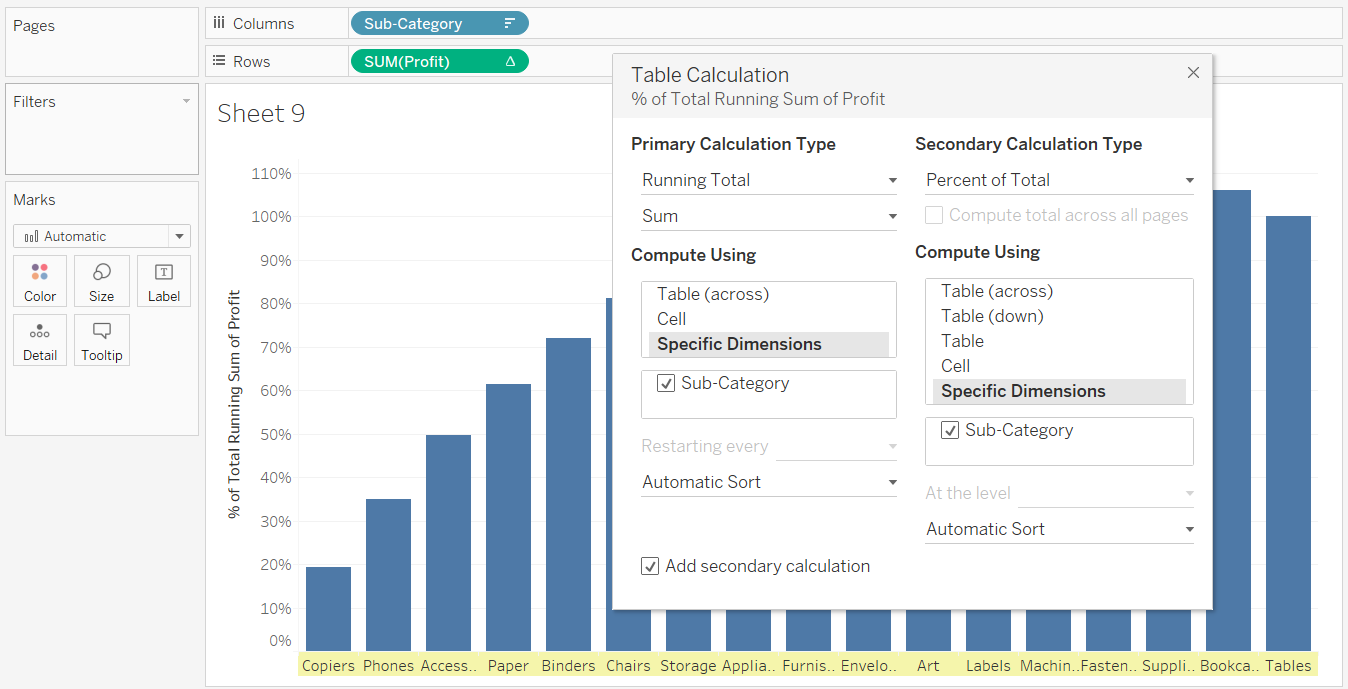Step 5: Analyze the Chart that you created. Pareto Chart is ready but the Analysis remains.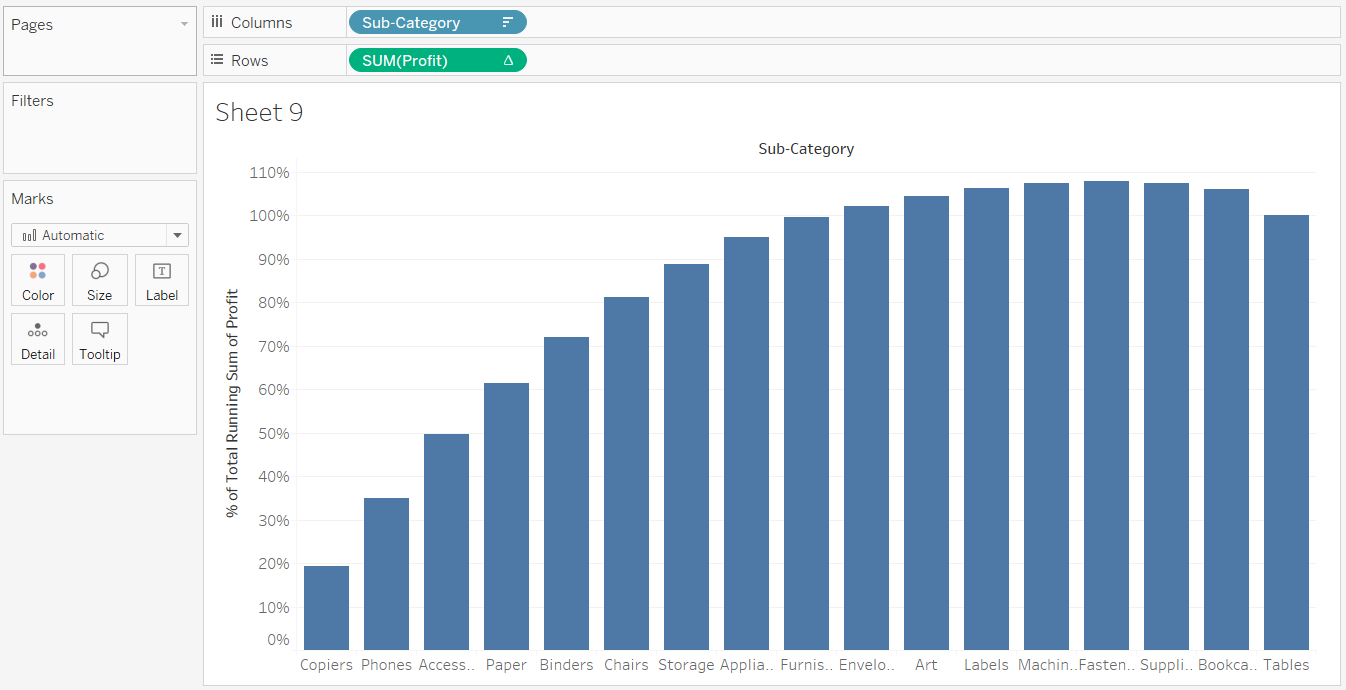So as we can see in the screen above, we can see the 80% of sales ( on the Y axis ) . And even without doing anything further we can see that 80% of sales on Y axis corresponds to 5 products of sub category i.e Copiers , Phones , Access, Paper , Binder, Chairs .

So out of total 17 Product Sub Category we have 6 product sub category that is reason for 80% of the profit and that makes 6/17 = 35% around. So we can say that Our Data does not follow the pareto principle as 80% of the Sales is being contributed by 35% of Product Sub Category and not 20% .

The Pareto Chart from Understanding perspective is complete, however if we want on the X- Axis instead of Product Sub Category Name we can show % so that, we can create trend lines of 80% on Y- Axis and 20% on X-axis to clearly see the 80-20 rule.

Now in order to calculate the % in x-axis , we have to do the same way as we calculate manually i.e. 6 / 17 i.e. index() / size () we will make a calculated field ParetoKPI and since we want to do it for “Sub Category” , we need to have the sub category in Marks shelf and compute Pareto KPI using “Sub Category”

Let’s do it in few steps as below

Step 6: To find out Percentage in x axis , create calculated field as ParetoKPI .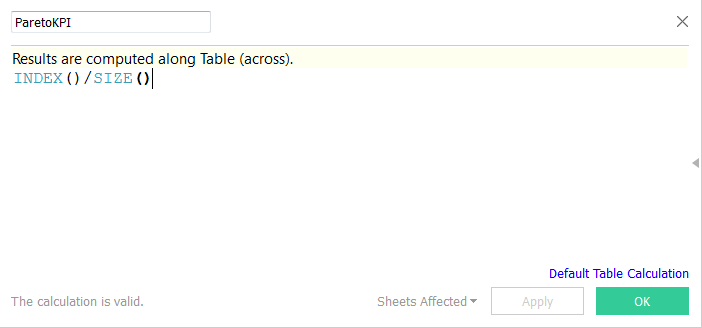Step 7: Thereafter Drag Sub Category from Columns to Mark Shelf and ParetoKPI to columns and for this Compute Using “Sub Category”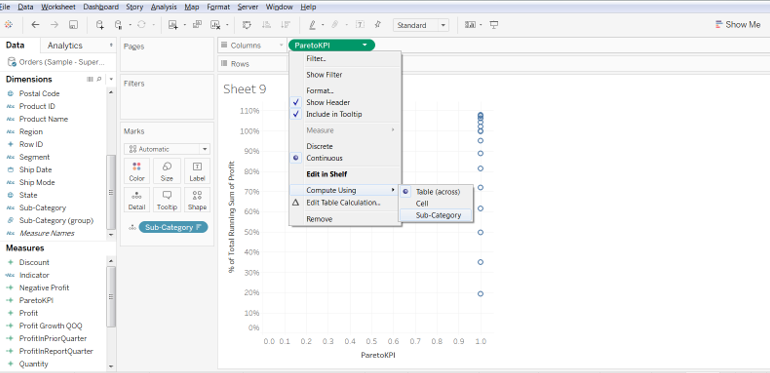Step 8:  In Marks Shelf change it to Line from Automatic and you get as below

So now we can see we have both Y axis and x axis in percent. We can format x axis to get in percentage.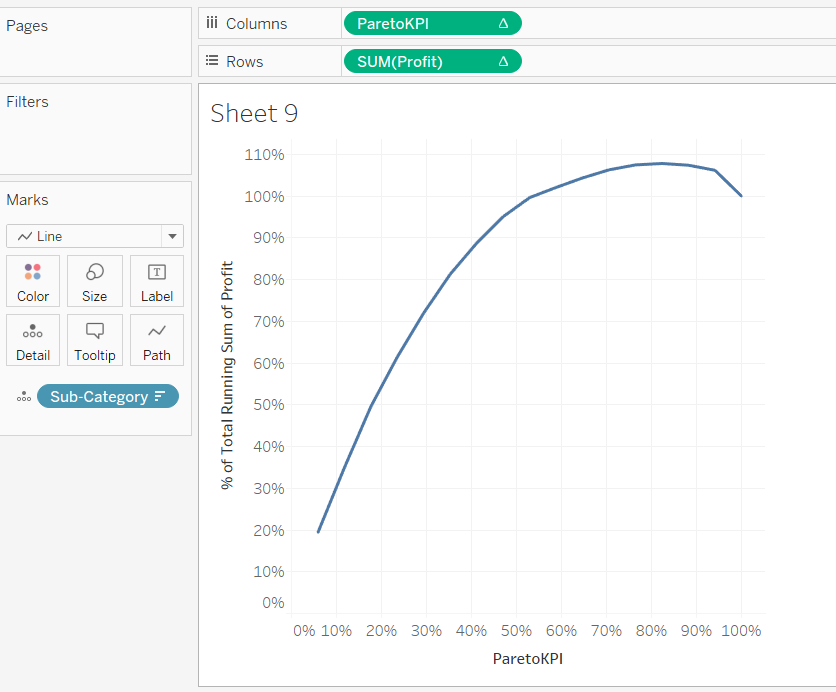Now we can drop 80% and 20% reference line on Yaxis and X axis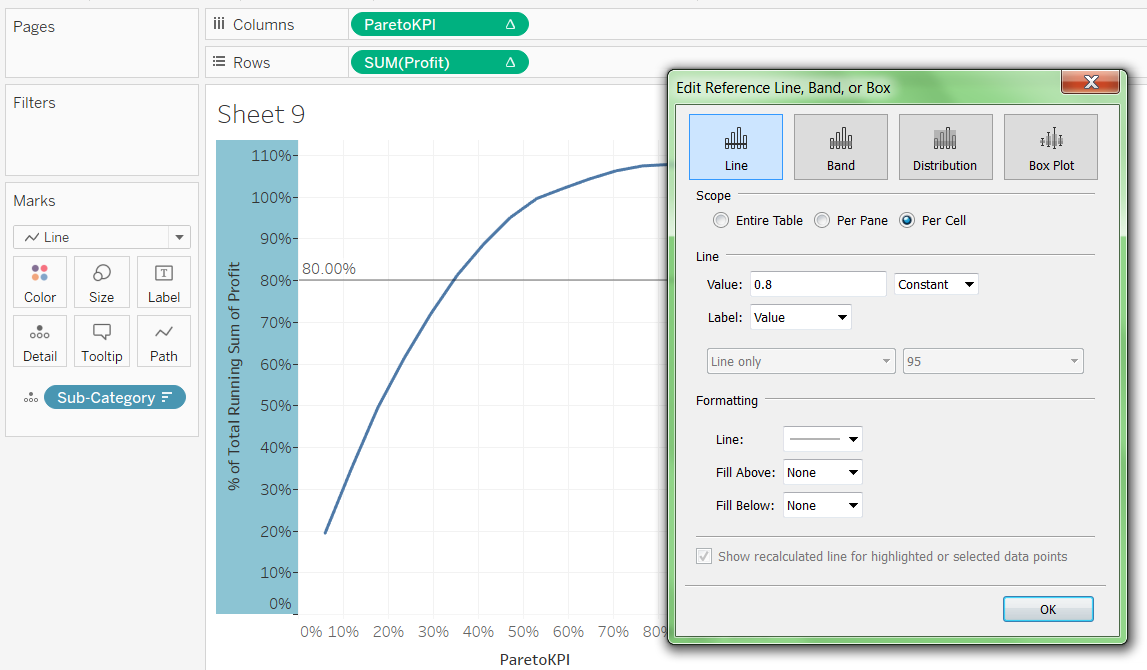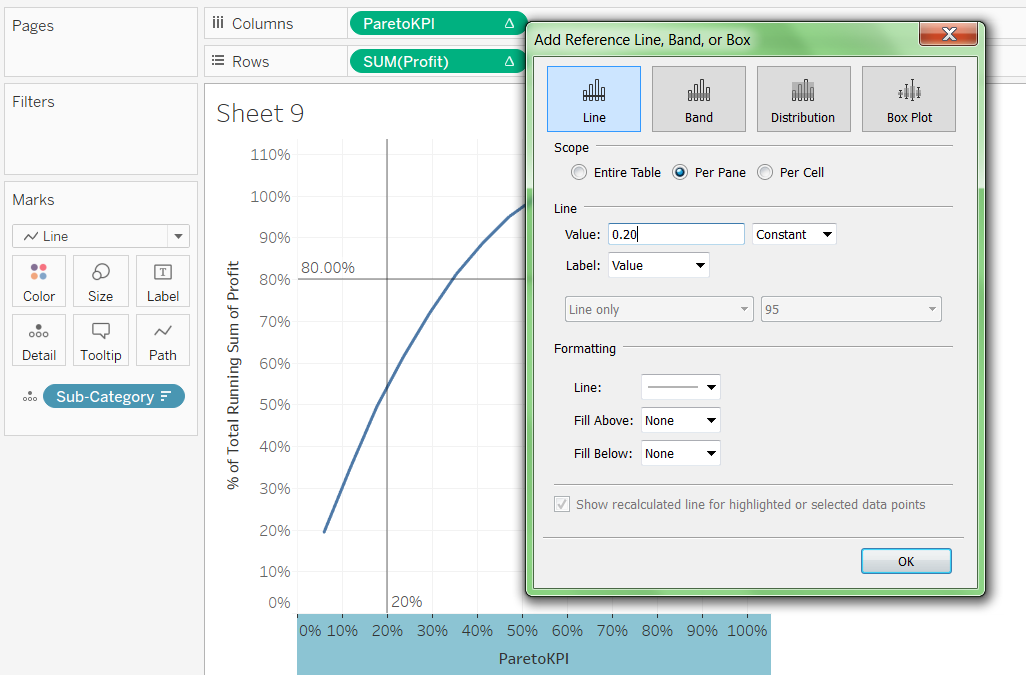So as we can see 20% of Product Sub Category leads to nearly 55% of the Profit. Since Sample Superstore is a mock up data , pareto principle does not satisfy. Even in real data ,whether Pareto principle satisfies or not depends on the How Data is distributed.